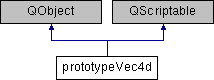Developer Documentation
prototypeVec4d Class Reference

`#include <OpenFlipper/Scripting/scriptPrototypes/prototypeVec4d.hh>`

Inheritance diagram for prototypeVec4d:## Public Slots

void multiply (QScriptValue _scalar)
Multiplies the given vector with the scalar. More...

Adds another vector to this vector. More...

void sub (QScriptValue _vector)
Subtracts another vector from this vector. More...

void zero ()
Resets all components of the vector to zero. More...

QScriptValue sprod (QScriptValue _vector)
Calculate scalar product. More...

QScriptValue norm ()
Calculate the Euclidean norm of the vector. More...

QScriptValue sqrnorm ()
Calculate the squared Euclidean norm of the vector. More...

void normalize ()
Normalize the vector.

QString toString () const

## Public Member Functions

prototypeVec4d (QObject *parent=0)

## Detailed Description

This class wraps the Vec4d to the scripting language

Definition at line 105 of file prototypeVec4d.hh.

## Member Function Documentation

 void prototypeVec4d::add ( QScriptValue _vector )
slot

Adds another vector to this vector.

Parameters
 _vector Vector that should be added

Definition at line 87 of file prototypeVec4d.cc.

 void prototypeVec4d::multiply ( QScriptValue _scalar )
slot

Multiplies the given vector with the scalar.

Parameters
 _scalar Scalar value that gets multiplied

Definition at line 80 of file prototypeVec4d.cc.

 QScriptValue prototypeVec4d::norm ( )
slot

Calculate the Euclidean norm of the vector.

Returns
Norm of the vector

Definition at line 117 of file prototypeVec4d.cc.

 QScriptValue prototypeVec4d::sprod ( QScriptValue _vector )
slot

Calculate scalar product.

Parameters
 _vector Second vector for scalar product (Can be the vector itself)
Returns
Scalar product value

Definition at line 109 of file prototypeVec4d.cc.

 QScriptValue prototypeVec4d::sqrnorm ( )
slot

Calculate the squared Euclidean norm of the vector.

Returns
Squared norm of the vector

Definition at line 124 of file prototypeVec4d.cc.

 void prototypeVec4d::sub ( QScriptValue _vector )
slot

Subtracts another vector from this vector.

Parameters
 _vector Vector that should be subtracted

Definition at line 95 of file prototypeVec4d.cc.

 void prototypeVec4d::zero ( )
slot

Resets all components of the vector to zero.

Definition at line 102 of file prototypeVec4d.cc.

The documentation for this class was generated from the following files: